### Choose language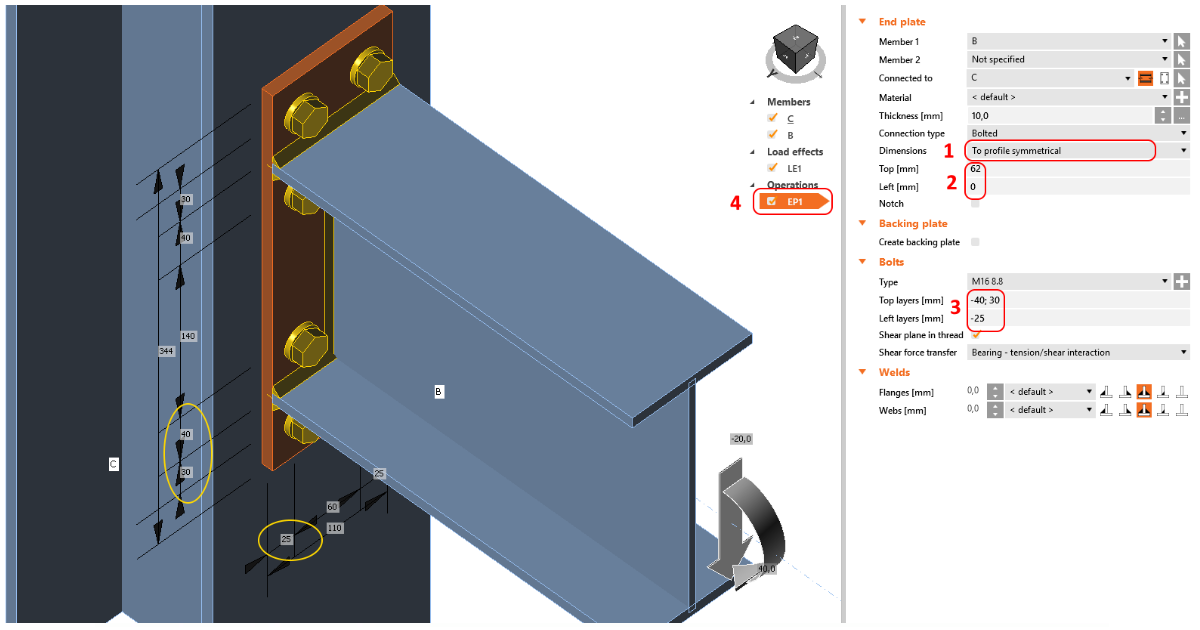# Bolts positioning in end plate operation

$$Let's take a look at few tips for how to input bolts in the End plate operation.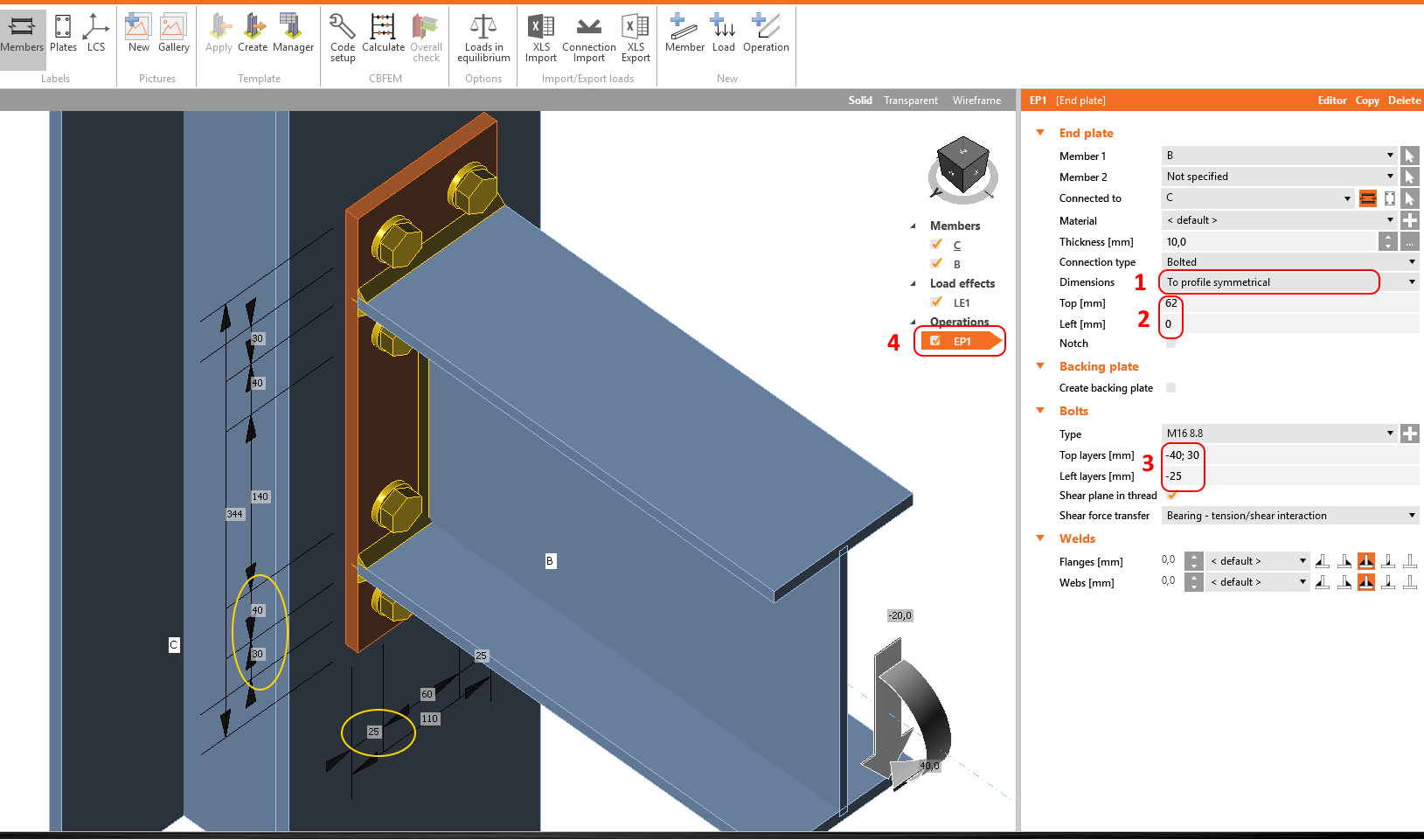First, set the type of the endplate.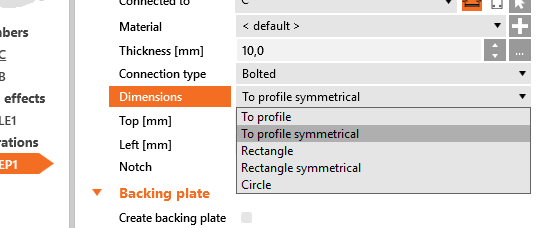And specify its dimensions. In this example, the dimensions are related to the outline of the connected beam cross-section.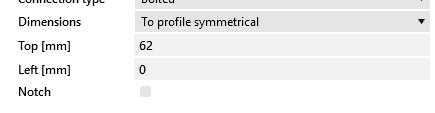Then input the bolt positions, filling in the values of layers.In this example, the bolts are symmetrical. The distance from the outline of the cross-section needs to be specified. A multiple number of rows and columns of bolts can be specified using delimiters. There are two types of delimiters which can be used for additional rows and columns:Absolute delimiter: ";" (a semi-colon)Relative delimiter: " " (space)If the semi-colon is used, every position is measured from the reference line (in this example, the outline of the cross-section).If space is used, the position of every other layer is measured from the previous one.Example: 40; 80; 120; 160; 200 equals to 40 40 40 40 40, or 4*40You can combine both delimiters: 40; 80; 120 40 40Example: The bolts ample can be specified in two ways with the same results: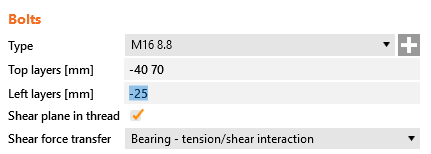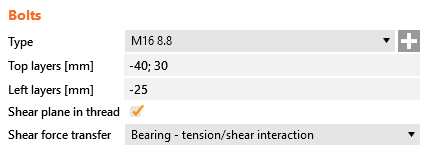To check the dimensions (bolt location, spacing, and edge distances), select the operation and the dimensions will be shown in the 3D scene.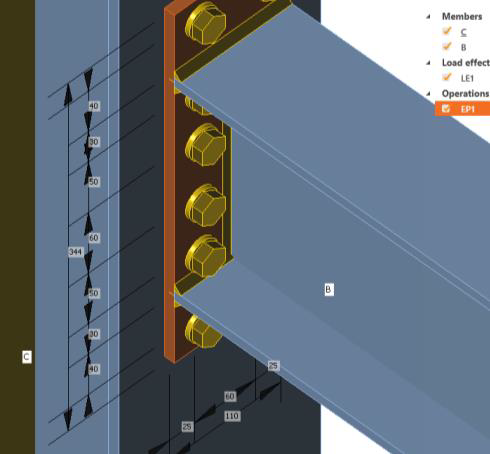$$